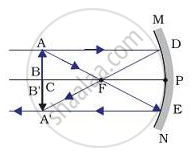# A spherical mirror produces an image of magnification -1.0 on a screen placed at a distance of 30 cm from the pole of the mirror - Science

A spherical mirror produces an image of magnification -1.0 on a screen placed at a distance of 30 cm from the pole of the mirror.

(i) Write the type of mirror in this case.

(ii) What is the focal length of the mirror ?

(iii) What is the nature of the image formed ?

(iv) Draw the ray diagram to show the image formation in this case.

#### Solution

Given :

m=-1 , v=-30 cm

We know that,

m=-v/u

therefore -1=(30)/u

therefore u=-30

(i) Since the magnification has a negative sign, the typa of mirror is a concave mirror

(ii) Using 1/f=1/v+1/u, we get

1/f=-1/30+(-1)/30

therefore 1/f=-2/30

therefore1/f=-1/15

=>f=-15 cm

∴ Focal length of the concave mirror is 15 cm

(iii) Since the magnitude of magnification is equal to 1 and the sign of magnification is negative, the image will be of the same size as the object, and its nature will be real and inverted.

(iv)Concept: Concave Mirror
Is there an error in this question or solution?
2013-2014 (March) Delhi Set 3

Share